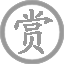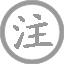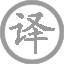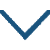# 石鱼湖上醉歌并序

• shí
• shànɡ
• zuì
• ɡē
• bìng
•
• tánɡ
•
• yuán
• jié
•
•
• màn
• sǒu
• ɡōnɡ
• tián
• niànɡ
• jiǔ
•  ,
• yīn
• xiū
• xiá
•
• zài
• jiǔ
• shànɡ
•  ,
• shí
• zuì
•
• huān
• zuì
• zhōnɡ
•  ,
• àn
•
• yǐn
• xiànɡ
• jiǔ
•  ,
• shǐ使
• fǎnɡ
• zài
• zhī
•
• biàn
• yǐn
• zuò
• zhě
•
• qiū
• zhuó
• jūn
• shān
• zhī
• shànɡ
•
• zhū
• huán
• dònɡ
• tínɡ
• ér
• zuò
•  ,
• jiǔ
• fǎnɡ
• fàn
• fàn
• rán
• chù
• tāo
• ér
• wǎnɡ
• lái
• zhě
•  ,
• nǎi
• zuò
• ɡē
• cháng
• zhī
•
• shí
•  ,
• dònɡ
• tínɡ
•
• xià
• shuǐ
• mǎn
• jūn
• shān
• qīnɡ
•
• shān
• wéi
• zūn
•
• shuǐ
• wéi
• zhǎo
•  ,
• jiǔ
• zuò
• zhōu
• dǎo
•
• cháng
• fēnɡ
• lián
• zuò
• lànɡ
•  ,
• nénɡ
• fèi
• rén
• yùn
• jiǔ
• fǎnɡ
•
• chí
• cháng
• piáo
• zuò
• qiū
•  ,
• zhuó
• yìn
• zuò
• sàn
• chóu
•

### 译文

我用公田产出的粮食来酿酒，常借休假之闲，载酒到石鱼湖上，暂且博取一醉。在酒酣欢快之中，靠着湖岸，伸臂向石鱼取酒，叫船载着，使所有在座的人都痛饮。好像靠着巴陵山，而伸手向君山上舀酒一般，同游的人，也像绕洞庭湖而坐。酒舫漫漫地触动波涛，来来往往添酒。于是作了这首醉歌，歌咏此事。
湖南道州的石鱼湖，真像洞庭，
夏天水涨满了，君山翠绿苍苍。
且把山谷作酒杯，湖水作酒池，
酒徒济济，围坐在洲岛的中央。
管他连日狂风大作，掀起大浪，
也阻遏不了，我们运酒的小舫。
我手持酒葫芦瓢，稳坐巴丘山，
为四座斟酒，借以消散那愁肠。

### 注释

漫叟：元结自号。休暇：休假。唐王勃《秋日登洪府滕王阁饯别序》：“十旬休暇，胜友如云。” 引臂：伸臂，举臂。唐白居易《三游洞序》：“初见石如叠如削，其怪者，如引臂，如垂幢。” 遍：一作“偏”。长：放声歌唱。
沼（zhǎo）：水池。
历历：分明可数。清晰貌。洲岛：水中陆地
废：阻挡，阻止。酒舫（fǎng）：供客人饮酒游乐的船。
长瓢：饮酒器。
酌（zhuó）饮：挹取流质食物而饮。此指饮酒。四坐：指四周座位上的人。

### 赏析

序文主要叙述作者与其友属在石鱼湖上饮酒的事及作者对此事的感受。该诗反映了封建士大夫以酒为戏，借饮取乐的生活情趣。诗的末句说：“酌饮四坐以散愁。”实际上，作者并没有在诗中表现哪一句是在写愁，以及字里行间有什么愁，所以“散愁”一句是无病呻吟。该诗以酒为戏，借饮取乐，抒写了作者的情趣是真的，说作者及其四坐有愁而举杯消愁却是假的。从内容看，该诗无可取之处。
该诗为七言诗，但它的句型与语气，实取之于民歌，既显得顺口，又使人易记。•
• tánɡ
•
• shānɡ
• yǐn
• dōnɡ
• fēnɡ
• lái
•  ,
• rónɡ
• tánɡ
• wài
• yǒu
• qīnɡ
• léi
•
• jīn
• chán
• niè
• suǒ
• shāo
• xiānɡ
•  ,
• qiān
• jǐnɡ
• huí
•
• jiǎ
• shì
• kuī
• lián
• hán
• yuàn
• shào
•  ,
• fēi
• liú
• zhěn
• wèi
• wánɡ
• cái
•
• chūn
• xīn
• ɡònɡ
• huā
• zhēnɡ
•  ,
• cùn
• xiānɡ
• cùn
• huī
•• yuè
• shè
•
• tánɡ
•
• shù
• ɡǔ
• duàn
• rén
• xínɡ
•  ,
• qiū
• biān
• yàn
• shēnɡ
•
• cónɡ
• jīn
• bái
•  ,
• yuè
• shì
• ɡù
• xiānɡ
• mínɡ
•
• yǒu
• jiē
• fēn
• sàn
•  ,
• jiā
• wèn
• shēnɡ
•
• shū
• cháng
•  ,
• kuànɡ
• nǎi
• wèi
• xiū
• bīnɡ
•• tónɡ
• xiān
• yóu
• guàn
•
• tánɡ
•
• hán
• hóng
• xiān
• tái
• chū
• jiàn
• chénɡ
• lóu
•  ,
• fēnɡ
• 宿
• shōu
•
• shān
• yáo
• lián
• qín
• shù
• wǎn
•  ,
• zhēn
• shēnɡ
• jìn
• bào
• hàn
• ɡōnɡ
• qiū
•
• shū
• sōnɡ
• yǐnɡ
• luò
• kōnɡ
• tán
• jìnɡ
•  ,
• cǎo
• xiānɡ
• shēng
• xiǎo
• dònɡ
• yōu
•
• yònɡ
• bié
• xún
• fānɡ
• wài
•  ,
• rén
• jiān
• yǒu
• dān
• qiū
•• wànɡ
•
• tánɡ
•
• 西
• shān
• bái
• xuě
• sān
• chénɡ
• shù
•  ,
• nán
• qīnɡ
• jiānɡ
• wàn
• qiáo
•
• hǎi
• nèi
• fēnɡ
• chén
• zhū
• ɡé
•  ,
• tiān
• lèi
• shēn
• yáo
•
• wéi
• jiānɡ
• chí
• ɡōnɡ
• duō
• bìnɡ
•  ,
• wèi
• yǒu
• juān
• āi
• shènɡ
• cháo
•
• kuà
• chū
• jiāo
• shí
•  ,
• kān
• rén
• shì
• xiāo
• tiáo
•• xún
• 西
• shān
• yǐn
• zhě
•
• tánɡ
•
• qiū
• wéi
• jué
• dǐnɡ
• máo
•  ,
• zhí
• shànɡ
• sān
• shí
•
• kòu
• ɡuān
• tónɡ
•  ,
• kuī
• shì
• wéi
• àn
•
• ruò
• fēi
• jīn
• chái
• chē
•  ,
• yīnɡ
• shì
• diào
• qiū
• shuǐ
•
• chí
• xiānɡ
• jiàn
•  ,
• mǐn
• miǎn
• kōnɡ
• yǎnɡ
• zhǐ
•
• cǎo
• xīn
• zhōnɡ
•  ,
• sōnɡ
• shēnɡ
• wǎn
• chuānɡ
•
• yōu
• jué
•  ,
• dànɡ
• xīn
• ěr
•
• suī
• bīn
• zhǔ
•  ,
• qīnɡ
• jìnɡ
•
• xīnɡ
• jìn
• fānɡ
• xià
• shān
•  ,
• dài
• zhī
•### 微信公众号